# plot

Plot probability distribution object

## Syntax

``plot(pd)``
``plot(ax,pd)``
``plot(___,Name=Value)``
``H = plot(___)``

## Description

example

````plot(pd)` plots a probability density function (pdf) of the probability distribution object `pd`. If `pd` is created by fitting a probability distribution to the data, the pdf is superimposed over a histogram of the data.```
````plot(ax,pd)` plots into the axes specified by the `Axes` graphics object `ax`.```

example

````plot(___,Name=Value)` specifies options using one or more name-value arguments in addition to any of the input argument combinations in the previous syntaxes. For example, you can indicate whether to plot a cumulative distribution function (cdf) or a probability plot instead of a pdf.```
````H = plot(___)` returns handles to the plotted graphics objects.```

## Examples

collapse all

Generate random data points from a normal distribution with mean 0 and standard deviation 1.

`rng("default") % Set the seed for reproducibility.`

Fit a normal distribution to the data.

```normaldata = normrnd(0,1,100,1); normalpd = fitdist(normaldata,"Normal")```
```normalpd = NormalDistribution Normal distribution mu = 0.123085 [-0.10756, 0.353731] sigma = 1.1624 [1.02059, 1.35033] ```

`normalpd` is a `NormalDistribution` object that contains the parameter values for the normal distribution fit to the data, and the data. Plot a pdf for the normal distribution with a histogram of the data.

`plot(normalpd)`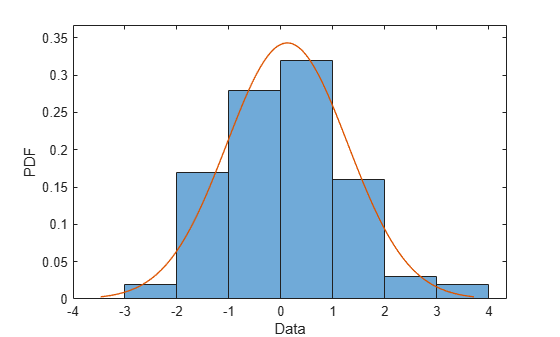Plot a cdf of the normal distribution fit to the data and a stairs plot of a cdf for the data.

`plot(normalpd,PlotType="cdf")`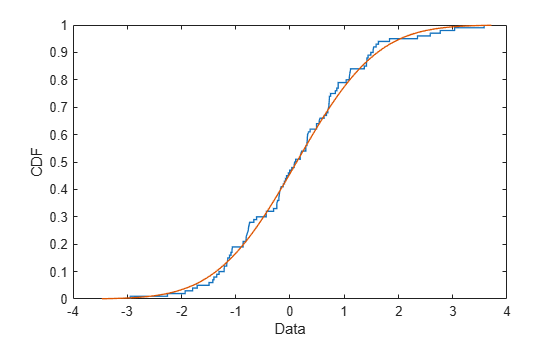Display a probability plot for the normal distribution fit to the data.

`plot(normalpd,PlotType="probability")`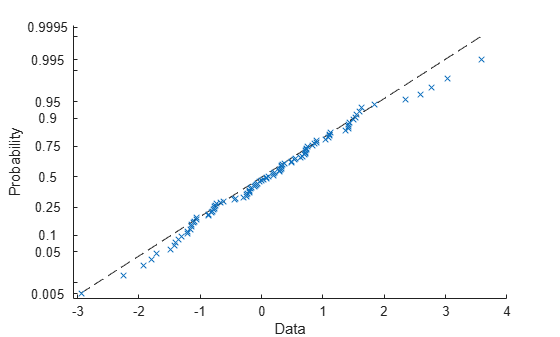The vertical axis is scaled so that the cdf for the fitted probability distribution is represented by a straight line.

Create a multinomial distribution that has five outcomes with probabilities of 0.1, 0.2, 0.4, 0.2, and 0.1.

`multinomialpd = makedist("Multinomial",probabilities=[0.1 0.2 0.4 0.2 0.1])`
```multinomialpd = MultinomialDistribution Probabilities: 0.1000 0.2000 0.4000 0.2000 0.1000 ```

Plot a pdf for the multinomial distribution.

`plot(multinomialpd)`The plot contains a `Stem` object that represents the probabilities for the data.

Plot the pdf as a continuous distribution.

`plot(multinomialpd,Discrete=0)`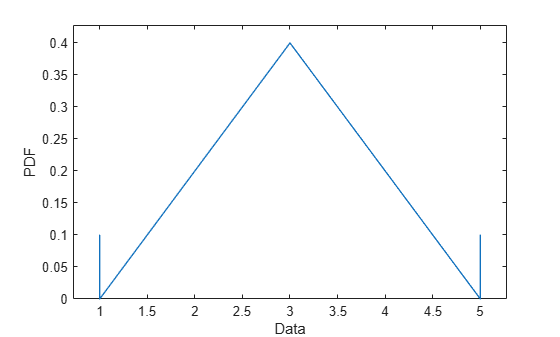Plot the cdf of the fitted multinomial distribution as a stairs plot.

`plot(multinomialpd,PlotType="cdf")`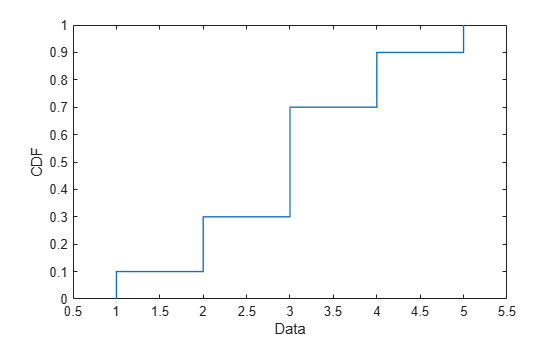Plot the cdf as a continuous distribution.

`plot(multinomialpd,PlotType="cdf",Discrete=0)`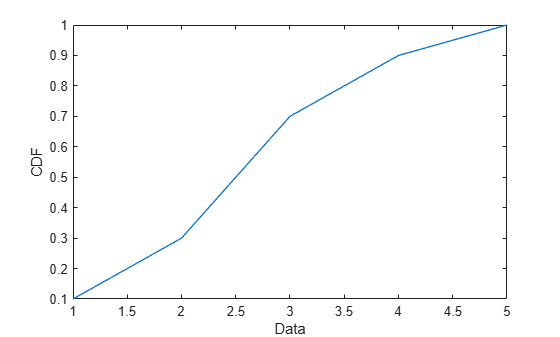## Input Arguments

collapse all

Probability distribution, specified as one of the probability distribution objects in the following table.

Distribution ObjectFunction or App Used to Create Probability Distribution Object
`BetaDistribution``makedist`, `fitdist`, Distribution Fitter
`BinomialDistribution``makedist`, `fitdist`, Distribution Fitter
`BirnbaumSaundersDistribution``makedist`, `fitdist`, Distribution Fitter
`BurrDistribution``makedist`, `fitdist`, Distribution Fitter
`ExponentialDistribution``makedist`, `fitdist`, Distribution Fitter
`ExtremeValueDistribution``makedist`, `fitdist`, Distribution Fitter
`GammaDistribution``makedist`, `fitdist`, Distribution Fitter
`GeneralizedExtremeValueDistribution``makedist`, `fitdist`, Distribution Fitter
`GeneralizedParetoDistribution``makedist`, `fitdist`, Distribution Fitter
`HalfNormalDistribution``makedist`, `fitdist`, Distribution Fitter
`InverseGaussianDistribution``makedist`, `fitdist`, Distribution Fitter
`KernelDistribution``fitdist`, Distribution Fitter
`LogisticDistribution``makedist`, `fitdist`, Distribution Fitter
`LoglogisticDistribution``makedist`, `fitdist`, Distribution Fitter
`LognormalDistribution``makedist`, `fitdist`, Distribution Fitter
`LoguniformDistribution``makedist`
`MultinomialDistribution``makedist`
`NakagamiDistribution``makedist`, `fitdist`, Distribution Fitter
`NegativeBinomialDistribution``makedist`, `fitdist`, Distribution Fitter
`NormalDistribution``makedist`, `fitdist`, Distribution Fitter
`PiecewiseLinearDistribution``makedist`
`PoissonDistribution``makedist`, `fitdist`, Distribution Fitter
`RayleighDistribution``makedist`, `fitdist`, Distribution Fitter
`RicianDistribution``makedist`, `fitdist`, Distribution Fitter
`StableDistribution``makedist`, `fitdist`, Distribution Fitter
`tLocationScaleDistribution``makedist`, `fitdist`, Distribution Fitter
`TriangularDistribution``makedist`
`UniformDistribution``makedist`
`WeibullDistribution``makedist`, `fitdist`, Distribution Fitter

Axes for plot, specified as an `Axes` graphics object. If you do not specify the axes by using the `ax` input argument or the `Parent` name-value argument, the `plot` function plots into the current axes or creates an `Axes` object if one does not exist. For more information on creating an `Axes` graphics object, see `axes` and Axes Properties.

### Name-Value Arguments

Specify optional pairs of arguments as `Name1=Value1,...,NameN=ValueN`, where `Name` is the argument name and `Value` is the corresponding value. Name-value arguments must appear after other arguments, but the order of the pairs does not matter.

Example: `Discrete=1,PlotType="cdf"` plots a cumulative distribution function (cdf) of the probability distribution as a stairs plot.

Plot type for the probability distribution, specified as one of the following.

 `"pdf"` Plot the probability density function (pdf). When `pd` is fit to data, the pdf is superimposed on a histogram of the data. `"cdf"` Plot the cumulative distribution function (cdf). When `pd` is fit to data, the cdf is superimposed over an empirical cdf. `"probability"` Display a probability plot using a cdf of the data and a cdf of the fitted probability distribution. This option is available only when `pd` is parametric and fit to data.

Example: `PlotType="probability"`

Data Types: `char` | `string`

Indicator to plot as a discrete function, specified as `1 (true)` or `0 (false)`. Specify `Discrete=0` to display the pdf or cdf as a line plot. Specify `Discrete=1` to display the pdf as a stem plot or to display the cdf as a stairs plot.

The default value for `Discrete` is 1 when `pd` is a discrete probability distribution object and 0 when `pd` is a continuous probability distribution object. If `pd` is continuous, `plot` ignores the user-specified input for `Discrete` and plots continuous functions.

Example: `Discrete=0`

Data Types: `logical`

Axes for plot, specified as an `Axes` graphics object. If you do not specify the axes by using the `ax` input argument or the `Parent` name-value argument, the `plot` function plots into the current axes or creates an `Axes` object if one does not exist. For more information on creating an `Axes` graphics object, see `axes` and Axes Properties.

## Output Arguments

collapse all

Handles to the plotted graphics objects, returned as a `Line` object, `Stem` object, `Stairs` object, or graphics array.

• When `PlotType` is set to `"pdf"` or `"cdf"`, and `pd` is not fit to data, `H` is a single handle corresponding to the pdf or cdf for the distribution.

• When `PlotType` is set to `"pdf"` or `"cdf"`, and `pd` is fit to data, `H` is a 1-by-2 graphics array. The first entry of the graphics array corresponds to the pdf or cdf for the distribution, and the second entry corresponds to the data.

• When `PlotType` is set to `"probability"`, `H` is a 2-by-1 graphics array with entries corresponding to the data and the distribution.

The table below shows how the returned graphics object depends on whether the distribution is discrete, whether `pd` is fit to data, and the specified values for the name-value arguments `Discrete` and `PlotType`.

Distribution TypeFit to Data`Discrete` value`PlotType` valueReturned Graphics Object
DiscreteNo1`"pdf"``Stem`
`"cdf"``Stairs`
0`"pdf"``Line`
`"cdf"``Line`
Yes1`"pdf"````[Stem Patch]```
`"cdf"````[Stairs Stairs]```
`"probability"````[Line FunctionLine]```
0`"pdf"````[Line Patch]```
`"cdf"````[Line Stairs]```
`"probability"````[Line FunctionLine]```
ContinuousNo0`"pdf"``Line`
`"cdf"``Line`
Yes0`"pdf"````[Line Histogram]```
`"cdf"````[Line Stairs]```
`"probability"````[Line FunctionLine]```

## Version History

Introduced in R2022b